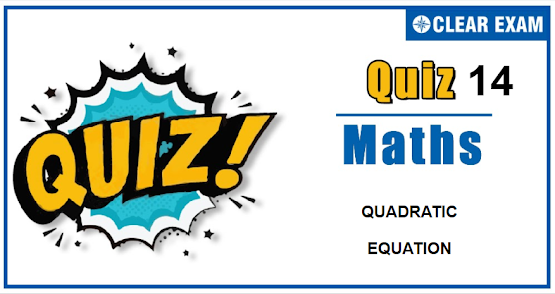## [LATEST]\$type=sticky\$show=home\$rm=0\$va=0\$count=4\$va=0

As per analysis for previous years, it has been observed that students preparing for JEE MAINS find Mathematics out of all the sections to be complex to handle and the majority of them are not able to comprehend the reason behind it. This problem arises especially because these aspirants appearing for the examination are more inclined to have a keen interest in Mathematics due to their ENGINEERING background.

Furthermore, sections such as Mathematics are dominantly based on theories, laws, numerical in comparison to a section of Engineering which is more of fact-based, Physics, and includes substantial explanations. By using the table given below, you easily and directly access to the topics and respective links of MCQs. Moreover, to make learning smooth and efficient, all the questions come with their supportive solutions to make utilization of time even more productive. Students will be covered for all their studies as the topics are available from basics to even the most advanced.

•  0
•  1
• -1
•  2
Solution
(b) Let each ratio be k and let A=xyz,
Then logx=k(a-b), logy=k(b-c)
And logz=k(c-a)
therefore logA=logx+logy+logz
=k(a-b)+k(b-c)+k(c-a)
=k[a-b+b-c+c-a]
=k
therefore logA=logxyz=0 [∵A=xyz]
⇒ xyz=e0=1

Q2.The solution set of x2+2 ≤ 3x ≤ 2x2-5, is
•  Φ
•  [1,2]
•  (-∞,1] U [5/2,∞)
•  None of these
Solution
(a) We have,
x2+2 ≤ 3x ≤ 2x2-5
⇒ x2-3x+2 ≤ 0 and 2x2-3x-5
≥ 0
⇒ (x-1)(x-2) ≤ 0 and (2x-5)(x+1) ≥0
⇒ 1   x ≤ 2 and x≤ -1 or x ≥ 5/2;
There is no value of x satisfying these conditions
Q3.  If one of the root of the equation x2+ax+3=0 is 3 and one of the roots of the equation x2+ax+b=0 is three times the other root, then the value of b is equal to
•  3
•  4
•  2
•  1
Q4. The value of the expression
1. (2-ω)(2-ω2)+2(3-ω)(3-ω2)+.........+(n-1)(n-ω)(n-ω2)
Where ω is an imaginary cube root of unit is
•  (n-1)n(n2+3n+4)/4
•  (n-1)n(n2+3n+4)/2
•  (n+1)n(n2+3n+4)/4
•  None of the above
Solution
Q6. If x2+px+q=0 has the roots α and β, then the value of (α-β)2 is equal to
•  p2-4q
• (p2-4q)2
•  p2+4q
• (p2+4q)2
Q8. Let x=αβ, y=αω+βω2, z=αω2+βω, ω is an imaginary cube root of unity. The value of xyz is
•  α22
•  α22
•  α33
•  α33
•  A square
•  A rectangle
•  A rhombus## Want to know more

Please fill in the details below:

## Latest NEET Articles\$type=three\$c=3\$author=hide\$comment=hide\$rm=hide\$date=hide\$snippet=hide

Name

ltr
item
BEST NEET COACHING CENTER | BEST IIT JEE COACHING INSTITUTE | BEST NEET, IIT JEE COACHING INSTITUTE: Quadratic_equation_Quiz-14